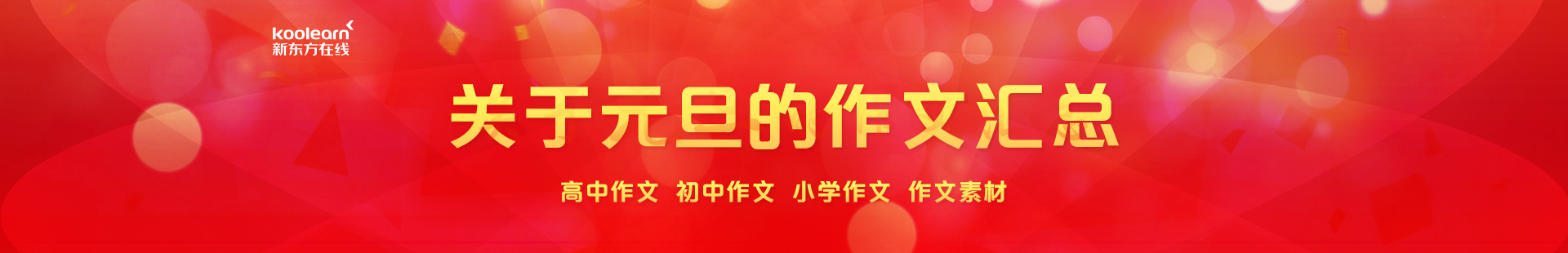```

```
`	`

```

```
`	`

```

```
`	`

```

```
`	`

```

```
`	`

```

```
`	`

```

```
`	`

```

```
`	`

```

```
`	`

```

```
`	`

```

```
`	`

```

```
`	`

```

```
`	`

```

```
`	`

```

```
`	`

```

```
`	`

```

```
`	`

```

```
`	`

```

```
`	`

```

```
`	`

```

```
`	`

```

```
`	`

```

```
`	`

```

```
`	`

```

```
`	`

```

```
`	`

```

```
`	`

```

```
`	`

```

```
`	`

```

```
`	`

```

```
`	`

```

```
`	`

```

```
`	`

```

```
`	`

```

```
`	`

```

```
`	`

```

```
`	`

```

```
`	`

```

```
`	`

```

```
`	`

```

```
`	`

```

```
`	`

```

```
`	`

```

```
`	`

```

```
`	`

```

```
`	`

```

```
`	`

```

```
`	`

```

```
`	`

```

```
`	`

```

```
`	`

```

```
`	`

```

```
`	`

```

```
`	`

```

```
`	`

```

```
`	`

```

```
`	`

```

```
`	`

```

```
`	`

```

```
`	`

```

```
`	`

```

```
`	`

```

```
`	`

```

```
`	`

```

```
`	`

```

```
`	`

```

```
`	`

```

```
`	`

```

```
`	`

```

```
`	`

```

```
`	`

```

```
`	`

```

```
`	`

```

```
`	`

```

```
`	`

```

```
`	`

```

```
`	`

```

```
`	`

```

```
`	`

```

```
`	`

```

```
`	`

```

```
`	`

```

```
`	`

```

```
`	`

```

```
`	`

```

```
`	`

```

```
`	`

```

```
`	`

```

```
`	`

```

```
`	`

```

```
`	`

```

```
`	`

```

```
`	`

```

```
`	`

```

```
`	`

```

```
`	`

```

```
`	`

```

```
`	`

```

```
`	`

```

```
`	`

```

```
`	`

```

```
`	`

```

```
`	`

```

```
`	`

```

```
`	`

```

```
`	`

```

```
`	`

```

```
`	`

```

```
`	`

```

```
`	`

```

```
`	`

```

```
`	`

```

```
`	`

```

```
`	`

```

```
`	`

```

```
`	`

```

```
`	`

```

```
`	`